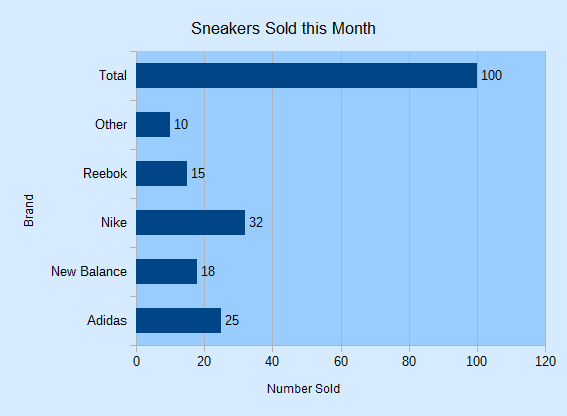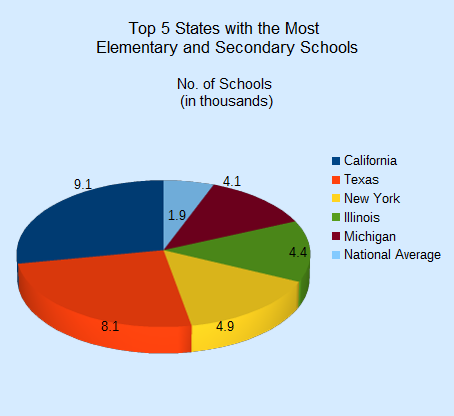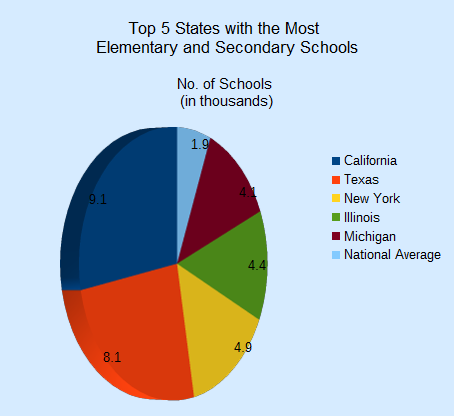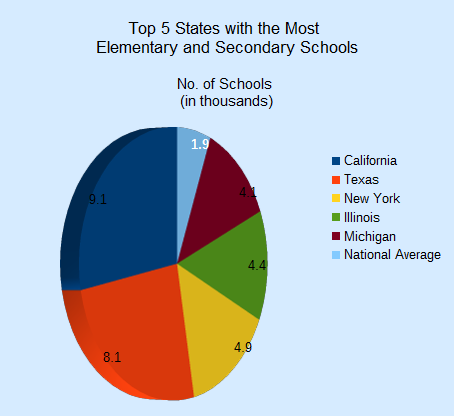# Chapter 30. Bar, Pie, Area, Line Charts

This chapter continues using the Chart2 Views example from the previous chapter, but looks at how bar, pie (including 3D and donut versions), area, and line charts can be generated from spreadsheet data. The relevant lines of chart_2_views.py are:

```# Chart2View.main() ofchart_2_views.py
def main(self) -> None:

try:
doc = Calc.open_doc(fnm=self._data_fnm)
GUI.set_visible(is_visible=True, odoc=doc)
sheet = Calc.get_sheet(doc=doc)

chart_doc = None
if self._chart_kind == ChartKind.AREA:
chart_doc = self._area_chart(doc=doc, sheet=sheet) # section 3
elif self._chart_kind == ChartKind.BAR:
chart_doc = self._bar_chart(doc=doc, sheet=sheet) # section 1
elif self._chart_kind == ChartKind.BUBBLE_LABELED:
chart_doc = self._labeled_bubble_chart(doc=doc, sheet=sheet)
elif self._chart_kind == ChartKind.COLUMN:
chart_doc = self._col_chart(doc=doc, sheet=sheet)
elif self._chart_kind == ChartKind.COLUMN_LINE:
chart_doc = self._col_line_chart(doc=doc, sheet=sheet)
elif self._chart_kind == ChartKind.COLUMN_MULTI:
chart_doc = self._mult_col_chart(doc=doc, sheet=sheet)
elif self._chart_kind == ChartKind.DONUT:
chart_doc = self._donut_chart(doc=doc, sheet=sheet) # 2.3
elif self._chart_kind == ChartKind.HAPPY_STOCK:
chart_doc = self._happy_stock_chart(doc=doc, sheet=sheet)
elif self._chart_kind == ChartKind.LINE:
chart_doc = self._line_chart(doc=doc, sheet=sheet) # section 4
elif self._chart_kind == ChartKind.LINES:
chart_doc = self._lines_chart(doc=doc, sheet=sheet) # section 4
elif self._chart_kind == ChartKind.NET:
chart_doc = self._net_chart(doc=doc, sheet=sheet)
elif self._chart_kind == ChartKind.PIE:
chart_doc = self._pie_chart(doc=doc, sheet=sheet)
elif self._chart_kind == ChartKind.PIE_3D:
chart_doc = self._pie_3d_chart(doc=doc, sheet=sheet) # section 2.1
elif self._chart_kind == ChartKind.SCATTER:
chart_doc = self._scatter_chart(doc=doc, sheet=sheet)
elif self._chart_kind == ChartKind.SCATTER_LINE_ERROR:
chart_doc = self._scatter_line_error_chart(doc=doc, sheet=sheet)
elif self._chart_kind == ChartKind.SCATTER_LINE_LOG:
chart_doc = self._scatter_line_log_chart(doc=doc, sheet=sheet)
elif self._chart_kind == ChartKind.STOCK_PRICES:
chart_doc = self._stock_prices_chart(doc=doc, sheet=sheet)

# ...
```

## 30.1 The Bar Chart

A bar chart is generated by `_bar_chart()` in chart_2_views.py using the “Sneakers Sold this Month” Table from Fig. 253.

The resulting chart is shown in Fig. 254.Fig. 254 :The Bar Chart for the Table in Fig. 253.

It’s informative to compare the bar chart in Fig. 254 with the column chart for the same data in Fig. 241. The data bars and axes have been swapped, so the `x-axis` in the column chart is the `y-axis` in the bar chart, and vice versa.

`_bar_chart()` is:

```# Chart2View._bar_chart() in chart_2_views.py
# uses "Sneakers Sold this Month" table
chart_doc = Chart2.insert_chart(
sheet=sheet,
cell_name="B3",
width=15,
height=11,
diagram_name=ChartTypes.Bar.TEMPLATE_STACKED.BAR,
)
Calc.goto_cell(cell_name="A1", doc=doc)

Chart2.set_title(
chart_doc=chart_doc, title=Calc.get_string(sheet=sheet, cell_name="A1")
)
Chart2.set_x_axis_title(
chart_doc=chart_doc, title=Calc.get_string(sheet=sheet, cell_name="A2")
)
Chart2.set_y_axis_title(
chart_doc=chart_doc, title=Calc.get_string(sheet=sheet, cell_name="B2")
)
# rotate x-axis which is now the vertical axis
Chart2.rotate_y_axis_title(chart_doc=chart_doc, angle=Angle(90))
return chart_doc
```

Although the axes have been swapped in the chart drawing, the API still uses the same indices to refer to the axes in `XCoordinateSystem.getAxisByDimension()`. This means that `x-axis` is the vertical axis in a bar chart, and `y-axis` the horizontal. This is most apparent in the last line of `_bar_chart()`:

```Chart2.rotate_y_axis_title(chart_doc=chart_doc, angle=Angle(90))
```

This causes the `x-axis` title to rotate 90 degrees counter-clockwise, which affects the `Brand` string on the vertical axis of the chart (see Fig. 254).

## 30.2 The Pie Chart

`_pie_chart()` in chart_2_views.py utilizes the “Top 5 States with the Most Elementary and Secondary Schools” table in `chartsData.ods` (see Fig. 255) to generate the pie chart in Fig. 256.

`_pie_chart()` is:

```# Chart2View._pie_chart() in chart_2_views.py
# uses "Top 5 States with the Most Elementary and Secondary Schools"
chart_doc = Chart2.insert_chart(
sheet=sheet,
cell_name="B10",
width=12,
height=11,
diagram_name=ChartTypes.Pie.TEMPLATE_DONUT.PIE,
)
Calc.goto_cell(cell_name="A1", doc=doc)

Chart2.set_title(
chart_doc=chart_doc, title=Calc.get_string(sheet=sheet, cell_name="E1")
)
Chart2.set_subtitle(
chart_doc=chart_doc, subtitle=Calc.get_string(sheet=sheet, cell_name="F2")
)
Chart2.view_legend(chart_doc=chart_doc, is_visible=True)
return chart_doc
```

`Chart2.set_subtitle()` displays the secondary heading in the chart; there’s little difference between it and the earlier `Chart2.set_title()`:

```# in Chart2 class
@classmethod
def set_subtitle(cls, chart_doc: XChartDocument, subtitle: str) -> XTitle:
try:
diagram = chart_doc.getFirstDiagram()
titled = Lo.qi(XTitled, diagram, True)
title = cls.create_title(subtitle)
titled.setTitleObject(title)
fname = Info.get_font_general_name()
cls.set_x_title_font(title, fname, 12)
return title
except ChartError:
raise
except Exception as e:
raise ChartError(f'Error setting subtitle "{subtitle}" for chart') from e
```

The XTitled reference for the subtitle is obtained from XDiagram, whereas the chart title is part of XChartDocument.

## 30.2.1 More 3D Pizzazz

For some reason people like 3D pie charts, so `_pie_3d_chart()` in chart_2_views.py produces one (see Fig. 257) using the same table as the 2D version.Fig. 257 :A 3D Pie Chart for the Table in Fig. 255.

`_pie_3d_chart()` is the same as `_pie_chart()` except that the `ThreeDPie` template is used:

```# Chart2View._pie_3d_chart() in chart_2_views.py
# uses "Top 5 States with the Most Elementary and Secondary Schools"
chart_doc = Chart2.insert_chart(
sheet=sheet,
cell_name="B10",
width=12,
height=11,
diagram_name=ChartTypes.Pie.TEMPLATE_3D.PIE_3D,
)
Calc.goto_cell(cell_name="A1", doc=doc)

Chart2.set_title(
chart_doc=chart_doc, title=Calc.get_string(sheet=sheet, cell_name="E1")
)
Chart2.set_subtitle(
chart_doc=chart_doc, subtitle=Calc.get_string(sheet=sheet, cell_name="F2")
)
Chart2.view_legend(chart_doc=chart_doc, is_visible=True)

# ...
# more code explained in a moment
```

The drawback of 3D pie charts is the shape distortion caused by the perspective. For example, the red segment in the foreground of Fig. 257 seems bigger than the dark blue segment at the back but that segment is numerical larger.

The default rotation of a 3D pie is `-60` degrees around the horizontal so its bottom edge appears to extend out of the page, and `0` degrees rotation around the vertical.

These can be changed by modifying the `RotationHorizontal` and `RotationVertical` properties of the Diagram service. For example:

```# part of Chart2View._pie_3d_chart() in chart_2_views.py
diagram = chart_doc.getFirstDiagram()
Props.set(
diagram,
RotationHorizontal=0,  # -ve rotates bottom edge out of page; default is -60
RotationVertical=-45,  # -ve rotates left edge out of page; default is 0 (i.e. no rotation)
)
```

This changes the pie chart’s appearance to be as in Fig. 258.Fig. 258 :A Rotated 3D Pie Chart for the Table in Fig. 255.

The easiest way to see the current values for the diagram’s properties is to add a call to `Props.show_obj_props()` to the code above:

```Props.show_obj_props("Diagram", diagram)
```

### 30.2.2 Changing the Data Point Labels

Two problems with Fig. 257 and Fig. 258 are the small data point labels and their default font color (black) which doesn’t stand out against the darker pie segments.

These issues can be fixed by changing some of the font related properties for the data points. This means a return to the DataSeries service shown in Fig. 259.

The DataPointProperties class appears twice in Fig. 259 because it allows the data point properties to be changed in two ways. The DataPointProperties class associated with the DataSeries service allows a property change to be applied to all the points collectively. The DataPointProperties class associated with a particular point allows a property to be changed only in that point.

For example, the former approach is used to change all the data point labels in the pie chart to `14pt`, `bold`, and `white`:

```# in Chart2View._pie_3d_chart() in chart_2_views.py
# ...
# change all the data points to be bold white 14pt
ds = Chart2.get_data_series(chart_doc)
Props.set(ds, CharHeight=14.0, CharColor=CommonColor.WHITE, CharWeight=FontWeight.BOLD)
#...
```

The changes to the chart are shown in Fig. 260.

The second approach is employed to emphasize the “National Average” data point label, which is the last one in the series:

```# end of Chart2View._pie_3d_chart() in chart_2_views.py
# ...
try:
props = Chart2.get_data_point_props(chart_doc=chart_doc, series_idx=0, idx=0)
Props.set(
props,
CharHeight=14.0,
CharColor=CommonColor.WHITE,
CharWeight=FontWeight.BOLD
)
except mEx.NotFoundError:
Lo.print("No Properties found for chart.")
return chart_doc
```

This produces the chart shown in Fig. 261, where only the National Average label is changed.Fig. 261 :A 3D Pie Chart with One Changed Data Point Label.

`Chart2.get_data_point_props()` takes three arguments - the chart document, the index of the data series, and the index of the data point inside that series. The pie chart uses six data points, so a valid index will be between `0` and `5`.

If a matching data point is found by `Chart2.get_data_point_props()` then a reference to its properties is returned, allowing that point to be modified:

```# in Chart2 class
@classmethod
def get_data_point_props(
cls, chart_doc: XChartDocument, series_idx: int, idx: int
) -> XPropertySet:
props = cls.get_data_points_props(chart_doc=chart_doc, idx=series_idx)
if not props:
raise NotFoundError("No Datapoints found to get XPropertySet from")

if idx < 0 or idx >= len(props):
raise IndexError(f"Index value of {idx} is out of of range")

return props[idx]
```

Also there is `Calc.get_data_points_props()` that takes two args and returns the properties for all the data points in a series:

```# in Chart2 class
@classmethod
def get_data_points_props(cls, chart_doc: XChartDocument, idx: int) -> List[XPropertySet]:
data_series_arr = cls.get_data_series(chart_doc=chart_doc)
if idx < 0 or idx >= len(data_series_arr):
raise IndexError(f"Index value of {idx} is out of of range")

props_lst: List[XPropertySet] = []
i = 0
while True:
try:
props = data_series_arr[idx].getDataPointByIndex(i)
if props is not None:
props_lst.append(props)
i += 1
except Exception:
props = None

if props is None:
break
if len(props_lst) > 0:
Lo.print(f"No Series at index {idx}")
return props_lst
```

`Chart2.get_data_series()` is called to get the data series for the chart type as a tuple.

This tuple is iterated over, collecting the property sets for each data point by calling `XDataSeries.getDataPointByIndex()`.

### 30.2.3 Anyone for Donuts?

If a table has more than one column of data then a `Donut` chart can be used to show each column as a ring.

`_donut_chart()` in chart_2_views.py utilizes the “Annual Expenditure on Institutions” table in `chartsData.ods` (see Fig. 262) to generate the donut chart with two rings in Fig. 263.

`_donut_chart()` is:

```# Chart2View._donut_chart() in chart_2_views.py
# uses the "Annual Expenditure on Institutions" table
chart_doc = Chart2.insert_chart(
sheet=sheet,
cell_name="D43",
width=15,
height=11,
diagram_name=ChartTypes.Pie.TEMPLATE_DONUT.DONUT,
)
Calc.goto_cell(cell_name="A48", doc=doc)

Chart2.set_title(chart_doc=chart_doc, title=Calc.get_string(sheet=sheet, cell_name="A43"))
Chart2.view_legend(chart_doc=chart_doc, is_visible=True)
subtitle = (
f'Outer: {Calc.get_string(sheet=sheet, cell_name="B44")}\n'
f'Inner: {Calc.get_string(sheet=sheet, cell_name="C44")}'
)
Chart2.set_subtitle(chart_doc=chart_doc, subtitle=subtitle)
return chart_doc
```

The only thing of note is the use of a more complex string for `Chart2.set_subtitle()` to display information about both rings.

## 30.3 The Area Chart

`_area_chart()` in chart_2_views.py utilizes the “Trends in Enrollment in Public Schools in the US” table in `chartsData.ods` (see Fig. 264) to generate the area chart in Fig. 265.

```# Chart2View._area_chart() in chart_2_views.py
# draws an area (stacked) chart;
# uses "Trends in Enrollment in Public Schools in the US" table
chart_doc = Chart2.insert_chart(
sheet=sheet,
cell_name="A52",
width=16,
height=11,
diagram_name=ChartTypes.Area.TEMPLATE_STACKED.AREA,
)
Calc.goto_cell(cell_name="A43", doc=doc)

Chart2.set_title(
chart_doc=chart_doc, title=Calc.get_string(sheet=sheet, cell_name="E43")
)
Chart2.set_x_axis_title(
chart_doc=chart_doc, title=Calc.get_string(sheet=sheet, cell_name="E45")
)
Chart2.set_y_axis_title(
chart_doc=chart_doc, title=Calc.get_string(sheet=sheet, cell_name="F44")
)
Chart2.view_legend(chart_doc=chart_doc, is_visible=True)
Chart2.rotate_y_axis_title(chart_doc=chart_doc, angle=Angle(90))
return chart_doc
```

If the `Area` template is replaced by `StackedArea` or `PercentStackedArea` then the two charts in Fig. 266 are generated.

## 30.4 The Line Chart

`_lines_chart()` in chart_2_views.py utilizes the “Trends in Expenditure Per Pupil” table in `chartsData.ods` (see Fig. 267) to generate two lines marked with symbols in Fig. 268.

`_lines_chart()` is:

```# Chart2View._lines_chart() in chart_2_views.py
# draw a line chart with data points, no legend;
# uses "Humidity Levels in NY" table
chart_doc = Chart2.insert_chart(
sheet=sheet,
cell_name="D13",
width=16,
height=9,
diagram_name=ChartTypes.Line.TEMPLATE_SYMBOL.LINE_SYMBOL,
)
Calc.goto_cell(cell_name="A1", doc=doc)

Chart2.set_title(
chart_doc=chart_doc, title=Calc.get_string(sheet=sheet, cell_name="A13")
)
Chart2.set_x_axis_title(
chart_doc=chart_doc, title=Calc.get_string(sheet=sheet, cell_name="A14")
)
Chart2.set_y_axis_title(
chart_doc=chart_doc, title=Calc.get_string(sheet=sheet, cell_name="B14")
)
```

`Chart2.set_data_point_labels()` switches off the displaying of the numerical data above the symbols so the chart is less cluttered.

There are many different line chart templates, as listed in Table 8. The `Line` template differs from `LineSymbol` by not including symbols over the data points.